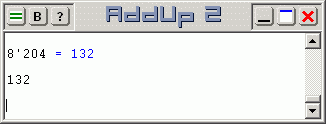# Octal Calculations with AddUp 2

Octal calculations are done by specifying a base-8 prefix for such numbers. Indeed, AddUp reads numbers expressed in any number base from 2 to 36. A number is in a base other than 10 when it is prefixed by its base value and a single quote. Base-2 (binary) numbers use a 2' prefix, base-16 (hexadecimal) numbers use an 16' prefix, base-3 numbers use a 3' prefix, and so on. For octal (base-8) numbers, this means using a 8' prefix before the number. So octal number 377 (decimal 255) is written as 8'377 while octal 123 (decimal 173) is written as 8'123.Output of all AddUp calculations is given in decimal unless specified otherwise. To explicitly ask for octal output, start an expression with a prefix similar to what is used in front of octal numbers: use '8 at the start of the line. Notice how the quote is on the left side of the requested output base. This line prefix must be followed by a space to separate it from the expression to evaluate. The expression to evaluate could either contain all octal numbers or a mixture of numbers in various numeric bases, but the result will be presented in the number base that the line prefix specifies. The default base-10 applies when no base is specified.